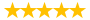# Week 5 Homework FIN540

Question # 00000335 Posted By: elliemay Updated on: 08/06/2013 03:06 PM Due on: 08/09/2013
Subject Economics Topic Financial Markets Tutorials:
Question

Week 5 Homework Assignment Chapter 23

Directions: Answer the following five questions on a separate document. Explain how you reached the answer or show your work if a mathematical calculation is needed, or both. Submit your assignment using the assignment link in the course shell. Each question is worth five points apiece for a total of 20 points for this homework assignment.

1. Which of the following statements about interest rate and reinvestment rate risk is CORRECT?

a. Variable (or floating) rate securities have more interest rate (price) risk than fixed rate securities.

b. Interest rate price risk exists because fixed-rate debt securities lose value when interest rates rise, while reinvestment rate risk is the risk of earning less than expected when interest payments or debt principal are reinvested.

c. Interest rate price risk can be eliminated by holding zero coupon bonds.

d. Reinvestment rate risk can be eliminated by holding variable (or floating) rate bonds.

e. Interest rate risk can never be reduced.

2. A commercial bank recognizes that its net income suffers whenever interest rates increase. Which of the following strategies would protect the bank against rising interest rates?

b. Entering into an interest rate swap where the bank receives a fixed payment stream, and in return agrees to make payments that float with market interest rates.

c. Purchase principal only (PO) strips that decline in value whenever interest rates rise.

d. Enter into a short hedge where the bank agrees to sell interest rate futures.

e. Sell some of the bank’s floating-rate loans and use the proceeds to make fixed-rate loans.

3. Suppose the September CBOT Treasury bond futures contract has a quoted price of 89-09. What is the implied annual interest rate inherent in this futures contract?

a. 6.32%

b. 6.65%

c. 7.00%

d. 7.35%

e. 7.72%

4. Suppose the December CBOT Treasury bond futures contract has a quoted price of 80-07. What is the implied annual interest rate inherent in the futures contract?

a. 6.86%

b. 7.22%

c. 7.60%

d. 8.00%

e. 8.40%

5. Suppose the December CBOT Treasury bond futures contract has a quoted price of 80-07. If annual interest rates go up by 1.00 percentage point, what is the gain or loss on the futures contract? (Assume a \$1,000 par value, and round to the nearest whole dollar.)

a. -\$78.00

b. -\$82.00

c. -\$86.00

d. -\$90.00

e. -\$95.00

Tutorials for this Question
1. ## Solution: Week 5 Homework Assignment Chapter 23 all answers correct

Tutorial # 00000229 Posted By: vikas Posted on: 08/06/2013 03:08 PM
Puchased By: 3
Tutorial Preview
is CORRECT?a. Variable (or floating) rate securities have more ...
Attachments
Week_5_Homework_Assignment_Chapter_23.docx (12.01 KB)
Recent Feedback
Rated By Feedback Comments Rated On
d...p82100% proofread tutorials 08/26/2014

Great! We have found the solution of this question!